``````##  "/private/var/folders/sc/6zzfzqbs5w7dl8q5g18tlqfc0000gn/T/Rtmp1KZtUn/Rinst1361973960727/TestGardener"
``````

# Introduction

In this vignette we run through an analysis of a typical rating scale, the Symptom Distress Scale used by the nursing profession to assess the level of distress of a hospitalized patient. The scale has a description of 13 distressing experiences, and the intensity or frequency of each is rated using the integers 0, 1, 2, 3 and 4. There are 473 respondents.

The vignette can serve as a template for the analysis of any rating scale or Likert type scale. It can also serve for tests scored using pre-assigned weights to assess the quality of the answer on something other than just “right/wrong.”

There are five distinct stages to the processing of the data: 1. Reading in the data. 2. Using the data to set up a named list object containing the information essential to their analysis. 3. The actual analysis 4. Producing desired graphs and other displays. 5. Simulating data in order to assess the accuracy of estimated quantities.

``````library(TestGardener)
#
# Attaching package: 'fda'
# The following object is masked from 'package:graphics':
#
#     matplot
``````

Here the data required for the analysis are entered. There are up to five objects to enter:

• a title for the analysis
• the choice data
• a character string for each item or question to display in plots
• a character string for each option choice within each item The two essential objects are the choice data and the right answer key. The other three are given default values.

The choice are an N by n matrix called `U` in the code. The rows of `U` correspond to examinees and the column to items or questions. Each number in `U` is the index of the option or answer that the examinee has chosen using the number 1 to `M` where `M` is the number of answers available for choice. The number of choices `M` can vary from one item to another. We call this data structure index mode data.

The choice indices may also be stored in a dataframe where all variables are of the factor type. We have avoided this level of sophistication since the only operations on `U` are summing and retrieving values and, given the potential size of `U`, it can be worthwhile to place additional restrictions on the structure such being in integer mode.

Note! Missing or illegal values in the matrix are treated as if they are an actual option or answer, and must be assigned the index `M` itself. If these are present, then the actual designed answers are numbered from 1 to `M-1`; otherwise `M` for an item is a proper answer choice. TestGardener treats missing or illegal choices in exactly the same way as it treats choices of actual answers, since whether or not an proper answer was chosen is itself information about the ability or status of the examinee.

Note again: The raw data provided to a test analyzer are often in what we call score mode where the value indicates the score or value assigned to that choice. This is often the case where the data are binary right/wrong values that are scored either 1 or 0, and is also often the case for rating scales. The test analyzer will have to convert these scores to indices before submitting `U` to TestGardener. In the binary data 1/0 case, this conversion just involves adding 1 to all the scores. The same is the case for rating scale scores that are 0 to some larger integer.

However, the data in the Symptom Distress Scale are already in index mode. Added to the five possible responses is a sixth choice for missing or illegal responses, of which there are many for all symptoms.

Finally, our example here is only one of many possible ways to construct a matrix `U`, since choice data can be read in from many types of files. The easiest case is the “.csv” file that consists of comma-separated values. Here we read in data supplied as rows of values not separated at all, and we treat these rows as single strings.

The `key` object is not needed for rating scale type questions, and we set it to NULL.

The reader may find it helpful to consult man pages for the functions used using the command `help("filename")` to obtain more detail and see example code.

First we will set up a title for the data to be used in plots:

``````titlestr  <- "Symptom Distress"
``````

We use the basic scan()' command to read the 473 lines in the file like this:

``````U         <- scan("SDS.txt","o")
# U         <- scan(paste(getwd(),"/data/SDS.txt",sep=""),"o")
U         <- matrix(U,473,2,byrow=TRUE)
U         <- U[,2]
N         <- length(U) # Number of examinees
Umat      <- as.integer(unlist(stringr::str_split(U,"")))
n         <- length(Umat)/N # Number of items
U         <- matrix(Umat,N,n,byrow=TRUE)
``````

We use the `stringr` package to break the each 13 character string into separate characters and then convert these characters to 13 integers. The result is an integer vector of length 13 times 6 with each set of 13 stacked on top of each other. The number of questions `n` is this length divided by the number of examinees.

However, we have also set up both `U` and `key` objects as .rda compressed files that are automatically loaded if `library(TestGardener)` has been entered.

There are many other ways in R to achieve the same result, of course, and this is unlikely to be viewed as the most elegant.

The right answer key is not needed, and is set to NULL here.

``````key <- NULL
``````

## Setting up the analysis

Now we turn to computing objects using these data that are essential to the analysis of the data.

All questions for these 24 questions in the test have four answers, but in case there are questions whether there are are missing or illegal answers for a question, we'll use this code to add the last wastebasket option where needed.

``````noption <- matrix(5,n,1)
for (i in 1:n)
{
if (any(U[,i] > noption[i]))
{
noption[i]  <- noption[i] + 1 # Add one option for invalid responses
U[U[,i] >= noption[i],i] <- noption[i]
}
}
``````

Now we turn to code that sets up objects that are essential to the analysis but that the analyst may want to set up using code rather than inputting. The first of these is a list vector of length `n` where each member contains a numeric vector of length `M` containing the designed scores assigned to each option. For multiple choice tests, that is easily done using the following code:

``````optScore <- list() # option scores
for (item in 1:n){
scorei <- c(0:4,0)
optScore[[item]] <- scorei
}
``````

There is also the possibility of inputting strings for each question and each option within each question. We won't use this feature in this analysis.

Here we define labels for the 13 forms of distress

``````itemVec <- c("Inability to sleep", "Fatigue", "Bowel symptoms", "Breathing symptoms",
"Coughing", "Inability to concentrate", "Intensity of nausea",
"Frequency of nausea", "Intensity of pain", "Frequency of pain",
"Bad outlook on life", "Loss of appetite", "Poor appearance")
``````

The ratings for each option, including “blank” for missing, are defined.

``````optVec <-c("0", "1", "2", "3", "4", " ")
optLab <- list()
for (i in 1:n)
{
optLab[[i]] = optVec
}
``````

Next, we set up a named list called `optList` that contains `ScoreList` and the item and option strings:

``````optList <- list(itemLab=itemVec, optLab=optLab, optScr=optScore)
``````

The maximum sum score possible would be 4 times 13 = 52. But in reality the highest sum score is only 37, but lots of people scored 0. We'll set for our plotting of sum scores and expected sums the upper limit at 37.

``````scrrng = c(0,37)
``````

We're now finished with the data input and setup phase. From these objects we have a function `make_dataList` that completes the set up:

``````SDS_dataList <- TestGardener::make.dataList(U, key, optList, scrrng=scrrng)
``````

The result is a named list with many members whose values may be required in the analysis phase. Consult the documentation using `help("make.dataList.R")`.

One default option requires a bit of explanation. Function `make.dataList()` computes sum scores for each examinee, which in the multiple choice case are simply counts of the number of correct answer choices. The analysis phase does not use these sum scores directly. Instead, it uses the rank orders of the sum scores (ordered from lowest to highest) divided by `N` and multipled by 100, so that the scores now look like percentages, and we refer to them as “percent ranks.”

A problem is that many examinees will get the same rank value if we order these ranks directly. We cannot know whether there might be some source of bias in how these tied ranks are arranged. For example, suppose male examinees for each rank value are followed by female examinees with that rank value. We deal with this problem by default by adding a small random normally distributed number to each tied rank that is too small to upset the original rank order. This within-rank randomization is called “jittering” in the statistics community, and its tends to break up the influence of any source of bias. This is the default, but can be turned off if desired.

These jittered percent ranks are used to provide initial values for a cycled optimization procedure used by TestGardener.

Let's make our first plot a display of the histogram and the probability density function for the sum scores.

``````hist(SDS_dataList\$scrvec, SDS_dataList\$scrrng, xlab="Sum Score",
main=titlestr)
``````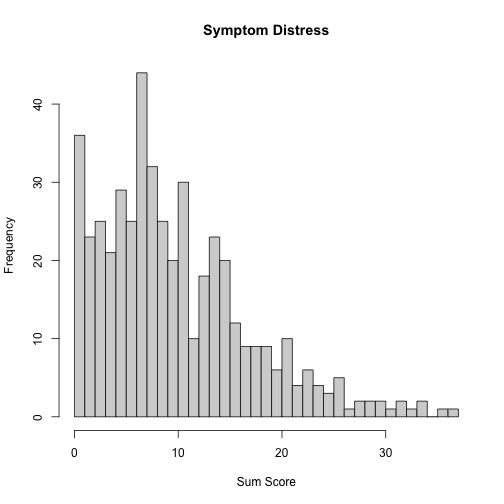We see that, on the whole, most patients do not experience great distress. A patient who rated all symptoms as 1 would be at about the 75% level, for example. The histogram indicates that the most popular score is 8, which is about half-and-half 0 and 1. The distribution is rather highly skewed in the positive direction.

From here on, the commands and text are essentially the same as those for the SweSAT math data, except for comments what we see in the figures.

The next steps are optional. The analysis can proceed without them, but the analyst may want to look at preliminary results that are associated with the the objects in the set up phase.

First we compute item response or item characteristic curves for each response within each question. These curves are historically probability curves, but we much prefer to work with a transformation of probability, “surprisal = - log(probability),” for two reasons: (1) this greatly aids the speed and accuracy of computing the curves since they are positive unbounded values, and (2) surprisal is in fact a measure of information, and as such has the same properties as other scientific measures. It's unit is the “bit” and what is especially important is that any fixed difference between two bit values means exactly the same thing everywhere on a surprisal curve.

The initializing of the surprisal curves is achieved as follows using the important function `Wbinsmth()`:

``````theta     <- SDS_dataList\$percntrnk
thetaQnt  <- SDS_dataList\$thetaQnt
chartList <- SDS_dataList\$chartList
WfdResult <- TestGardener::Wbinsmth(theta, SDS_dataList, thetaQnt, chartList)
``````

One may well want to plot these initial surprisal curves in terms of both probability and surprisal, this is achieved in this way:

``````WfdList <- WfdResult\$WfdList
binctr  <- WfdResult\$aves
Qvec    <- c(5,25,50,75,95)
TestGardener::Wbinsmth.plot(binctr, Qvec, WfdList, SDS_dataList, Wrng=c(0,3), plotindex=1)
``````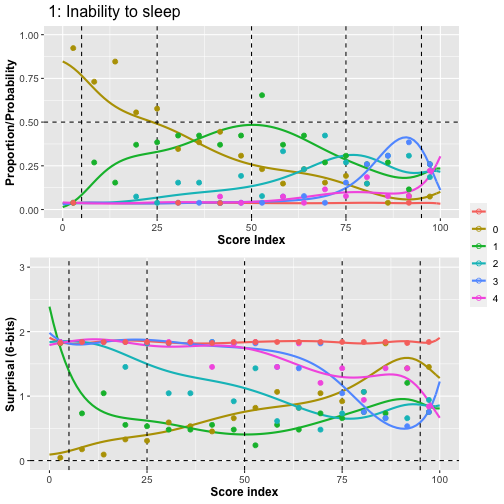Here here and elsewhere we only plot the probability and surprisal curves for the first time in order to allow this vignette to be displayed compactly. There are comments on how to interpret these plots in the call to function `Wbinsmth.plot()` after the analysis step.

## Cycling through the estimation steps

Our approach to computing optimal estimates of surprisal curves and examinee percentile rank values involves alternating between:

1. estimating surprisal curves assuming the previously computed percentile ranks are known
2. estimating examinee percentile ranks assuming the surprisal curves are known.

This is a common strategy in statistics, and especially when results are not required to be completely optimal. We remind ourselves that nobody would need a test score that is accurate to many decimal places. One decimal place would surely do just fine from a user's perspective.

We first choose a number of cycles that experience indicates is sufficient to achieve nearly optimal results, and then at the end of the cycles we display a measure of the total fit to the data for each cycle as a check that sufficient cycles have been carried. Finally, we choose a cycle that appears to be sufficiently effective. A list object is also defined that contains the various results at each cycle.

In this case the measure of total fit is the average of the fitting criterion across all examinees. The fitting criterion for each examinee is the negative of the log of the likelihood, or maximum likelihood estimation. Here we choose 10 cycles.

``````ncycle <- 10
``````

Here is a brief description of the steps within each cycle

### Step 1: Bin the data, and smooth the binned data to estimate surprisal curves

Before we invoke function `Wbinsmth`, we use the first three lines to define bin boundaries and centres so as to have a roughly equal number of examinees in each bin. Vector `denscdfi` has already been set up that contains values of the cumulative probability distribution for the percentile ranks at a fine mesh of discrete points. Bin locations and the locations of the five marker percents in `Qvec` are set up using interpolation. Surprisal curves are then estimated and the results set up.

### Step 2: Compute optimal score index values

Function `thetafun()` estimates examinee percentile values given the curve shapes that we've estimated in Step 1. The average criterion value is also computed and stored.

### Step 3: Estimate the percentile rank density

The density that we need is only for those percentile ranks not located at either boundary, function `theta.distn()` only works with these. The results will be used in the next cycle.

### Step 4: Estimate arc length scores along the test effort curve

The test information curve is the curve in the space of dimension `Wdim` that is defined by the evolution of all the curves jointly as their percentile index values range from 0 to 100%.

### Step 5: Set up the list object that stores results

All the results generated in this cycle are bundled together in a named list object for access at the end of the cycles. The line saves the object in the list vector `SDS_dataResult`.

Here is the single command that launches the analysis:

``````AnalyzeResult <- TestGardener::Analyze(theta, thetaQnt, SDS_dataList, ncycle=ncycle, itdisp=TRUE)
#  "Cycle  1"
#  "Mean surprisal =  7.67"
#  "Number of nonpositive D2H = 94"
#  "mean adjusted values = 47.36"
#  "iter 1 ,  nactive =  470"
#  "iter 2 ,  nactive =  142"
#  "iter 3 ,  nactive =  30"
#  "iter 4 ,  nactive =  3"
#  "arclength in bits =  19.9"
#  "Cycle  2"
#  "Mean surprisal =  7.268"
#  "Number of nonpositive D2H = 76"
#  "mean adjusted values = 47.37"
#  "iter 1 ,  nactive =  459"
#  "iter 2 ,  nactive =  166"
#  "iter 3 ,  nactive =  64"
#  "iter 4 ,  nactive =  23"
#  "iter 5 ,  nactive =  11"
#  "iter 6 ,  nactive =  5"
#  "iter 7 ,  nactive =  2"
#  "iter 8 ,  nactive =  2"
#  "iter 9 ,  nactive =  1"
#  "iter 10 ,  nactive =  1"
#  "iter 11 ,  nactive =  1"
#  "iter 12 ,  nactive =  1"
#  "iter 13 ,  nactive =  1"
#  "iter 14 ,  nactive =  1"
#  "iter 15 ,  nactive =  1"
#  "iter 16 ,  nactive =  1"
#  "iter 17 ,  nactive =  1"
#  "iter 18 ,  nactive =  1"
#  "iter 19 ,  nactive =  1"
#  "iter 20 ,  nactive =  1"
#  "arclength in bits =  29.5"
#  "Cycle  3"
#  "Mean surprisal =  7.193"
#  "Number of nonpositive D2H = 57"
#  "mean adjusted values = 41.89"
#  "iter 1 ,  nactive =  461"
#  "iter 2 ,  nactive =  165"
#  "iter 3 ,  nactive =  67"
#  "iter 4 ,  nactive =  34"
#  "iter 5 ,  nactive =  15"
#  "iter 6 ,  nactive =  9"
#  "iter 7 ,  nactive =  7"
#  "iter 8 ,  nactive =  6"
#  "iter 9 ,  nactive =  4"
#  "iter 10 ,  nactive =  2"
#  "iter 11 ,  nactive =  1"
#  "iter 12 ,  nactive =  1"
#  "iter 13 ,  nactive =  1"
#  "iter 14 ,  nactive =  1"
#  "iter 15 ,  nactive =  1"
#  "iter 16 ,  nactive =  1"
#  "iter 17 ,  nactive =  1"
#  "iter 18 ,  nactive =  1"
#  "iter 19 ,  nactive =  1"
#  "iter 20 ,  nactive =  1"
#  "arclength in bits =  33.9"
#  "Cycle  4"
#  "Mean surprisal =  7.11"
#  "Number of nonpositive D2H = 38"
#  "mean adjusted values = 44.53"
#  "iter 1 ,  nactive =  467"
#  "iter 2 ,  nactive =  157"
#  "iter 3 ,  nactive =  70"
#  "iter 4 ,  nactive =  44"
#  "iter 5 ,  nactive =  19"
#  "iter 6 ,  nactive =  14"
#  "iter 7 ,  nactive =  6"
#  "iter 8 ,  nactive =  4"
#  "iter 9 ,  nactive =  1"
#  "iter 10 ,  nactive =  1"
#  "iter 11 ,  nactive =  1"
#  "arclength in bits =  37.9"
#  "Cycle  5"
#  "Mean surprisal =  7.095"
#  "Number of nonpositive D2H = 28"
#  "mean adjusted values = 50.86"
#  "iter 1 ,  nactive =  467"
#  "iter 2 ,  nactive =  123"
#  "iter 3 ,  nactive =  63"
#  "iter 4 ,  nactive =  30"
#  "iter 5 ,  nactive =  13"
#  "iter 6 ,  nactive =  7"
#  "iter 7 ,  nactive =  5"
#  "iter 8 ,  nactive =  2"
#  "iter 9 ,  nactive =  2"
#  "iter 10 ,  nactive =  2"
#  "iter 11 ,  nactive =  2"
#  "iter 12 ,  nactive =  2"
#  "iter 13 ,  nactive =  2"
#  "iter 14 ,  nactive =  2"
#  "iter 15 ,  nactive =  1"
#  "arclength in bits =  37.1"
#  "Cycle  6"
#  "Mean surprisal =  7.087"
#  "Number of nonpositive D2H = 18"
#  "mean adjusted values = 50.22"
#  "iter 1 ,  nactive =  468"
#  "iter 2 ,  nactive =  108"
#  "iter 3 ,  nactive =  56"
#  "iter 4 ,  nactive =  33"
#  "iter 5 ,  nactive =  16"
#  "iter 6 ,  nactive =  6"
#  "iter 7 ,  nactive =  3"
#  "iter 8 ,  nactive =  1"
#  "iter 9 ,  nactive =  1"
#  "iter 10 ,  nactive =  1"
#  "iter 11 ,  nactive =  1"
#  "iter 12 ,  nactive =  1"
#  "iter 13 ,  nactive =  1"
#  "iter 14 ,  nactive =  1"
#  "iter 15 ,  nactive =  1"
#  "iter 16 ,  nactive =  1"
#  "iter 17 ,  nactive =  1"
#  "iter 18 ,  nactive =  1"
#  "arclength in bits =  37.8"
#  "Cycle  7"
#  "Mean surprisal =  7.072"
#  "Number of nonpositive D2H = 14"
#  "mean adjusted values = 54.29"
#  "iter 1 ,  nactive =  469"
#  "iter 2 ,  nactive =  122"
#  "iter 3 ,  nactive =  62"
#  "iter 4 ,  nactive =  27"
#  "iter 5 ,  nactive =  19"
#  "iter 6 ,  nactive =  12"
#  "iter 7 ,  nactive =  7"
#  "iter 8 ,  nactive =  6"
#  "iter 9 ,  nactive =  5"
#  "iter 10 ,  nactive =  2"
#  "iter 11 ,  nactive =  2"
#  "iter 12 ,  nactive =  1"
#  "iter 13 ,  nactive =  1"
#  "iter 14 ,  nactive =  1"
#  "iter 15 ,  nactive =  1"
#  "iter 16 ,  nactive =  1"
#  "iter 17 ,  nactive =  1"
#  "iter 18 ,  nactive =  1"
#  "iter 19 ,  nactive =  1"
#  "iter 20 ,  nactive =  1"
#  "arclength in bits =  38.3"
#  "Cycle  8"
#  "Mean surprisal =  7.063"
#  "Number of nonpositive D2H = 12"
#  "mean adjusted values = 51.67"
#  "iter 1 ,  nactive =  468"
#  "iter 2 ,  nactive =  79"
#  "iter 3 ,  nactive =  44"
#  "iter 4 ,  nactive =  28"
#  "iter 5 ,  nactive =  20"
#  "iter 6 ,  nactive =  13"
#  "iter 7 ,  nactive =  5"
#  "iter 8 ,  nactive =  2"
#  "iter 9 ,  nactive =  2"
#  "iter 10 ,  nactive =  2"
#  "iter 11 ,  nactive =  2"
#  "iter 12 ,  nactive =  1"
#  "iter 13 ,  nactive =  1"
#  "iter 14 ,  nactive =  1"
#  "arclength in bits =  38"
#  "Cycle  9"
#  "Mean surprisal =  7.066"
#  "Number of nonpositive D2H = 9"
#  "mean adjusted values = 41.78"
#  "iter 1 ,  nactive =  468"
#  "iter 2 ,  nactive =  70"
#  "iter 3 ,  nactive =  37"
#  "iter 4 ,  nactive =  25"
#  "iter 5 ,  nactive =  11"
#  "iter 6 ,  nactive =  8"
#  "iter 7 ,  nactive =  4"
#  "iter 8 ,  nactive =  3"
#  "iter 9 ,  nactive =  2"
#  "arclength in bits =  36.8"
#  "Cycle  10"
#  "Mean surprisal =  7.072"
#  "Number of nonpositive D2H = 7"
#  "mean adjusted values = 58.29"
#  "iter 1 ,  nactive =  465"
#  "iter 2 ,  nactive =  87"
#  "iter 3 ,  nactive =  47"
#  "iter 4 ,  nactive =  26"
#  "iter 5 ,  nactive =  13"
#  "iter 6 ,  nactive =  9"
#  "iter 7 ,  nactive =  5"
#  "iter 8 ,  nactive =  3"
#  "iter 9 ,  nactive =  2"
#  "iter 10 ,  nactive =  2"
#  "iter 11 ,  nactive =  2"
#  "iter 12 ,  nactive =  2"
#  "iter 13 ,  nactive =  2"
#  "iter 14 ,  nactive =  2"
#  "iter 15 ,  nactive =  2"
#  "iter 16 ,  nactive =  1"
#  "arclength in bits =  36.9"
``````

The following two lines set up a list vector object of length 10 containing the results on each cycle, and also a numeric vector of the same length containing averages of the fitting criterion values for each examinee.

``````parList  <- AnalyzeResult\$parList
meanHvec <- AnalyzeResult\$meanHvec
``````

## Displaying the results of the analysis cycles

### Plot `meanHsave` and choose cycle for plotting

The mean fitting values in `meanHvec` should decrease, and then level off as we approach optimal estimations of important model objects, such as optimal percent ranks in numeric vector `theta` and optimal surprisal curves in list vector `WfdList`. Plotting these values as a function of cycle number will allow us to choose a best cycle for displaying results.

``````cycleno <- 1:ncycle
plot(cycleno,meanHvec[cycleno], type="b", lwd=2, xlab="Cycle Number")
``````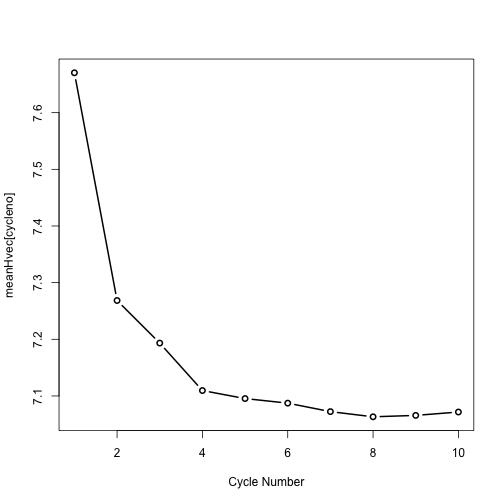This plot shows a nice exponential-like decline in the average fitting criterion `meanHvec` over ten iterations. It does look like we could derive a small benefit from a few more iterations, but the changes in what we estimate using super precision in the minimization will be too small to be of any practical interest.

Here we choose to display results for the last cycle:

``````icycle <- 10
SDS_parListi  <- parList[[icycle]]
``````

The list object `SDS_parListi` contains a large number of objects, but in our exploration of results, we will only need these results:

``````WfdList    <- SDS_parListi\$WfdList
theta      <- SDS_parListi\$theta
Qvec       <- SDS_parListi\$Qvec
binctr     <- SDS_parListi\$binctr
arclength  <- SDS_parListi\$arclength
alfine     <- SDS_parListi\$alfine
``````
• `WfdList`: List object of length `n` that contains information about the surprisal and probability curves
• `theta`: A vector of length N of values of what we call “score index”, which are real numbers between 0 and 100. These values are used for computing various score values such as expected sum scores.
• `Qvec`: Values within the range 0 to 100 below which these percentages of examinees fall: 5, 25, 50, 75 and 95.
• `binctr`: The surprisal curves are estimated by first binning the values in vector `theta` and then using the surprisal values within each bin. `binctr` contains the values at the centres of the bins.
• `arclength`: This is the total length of the curve traced out by the surprisal scores as `theta` moves from 0 to 100. `alfine`: An equally spaced set of values along this space curved, used for plotting purposes.

### Plot surprisal curves for test questions

Well, here we just plot the probability and surprisal curves for just symptom eight as an illustration, namely `Frequency of nausea`. If argument `plotindex` is omitted, curves for all questions would be plotted.

``````TestGardener::Wbinsmth.plot(binctr, Qvec, WfdList, SDS_dataList, Wrng=c(0,3), plotindex=8)
``````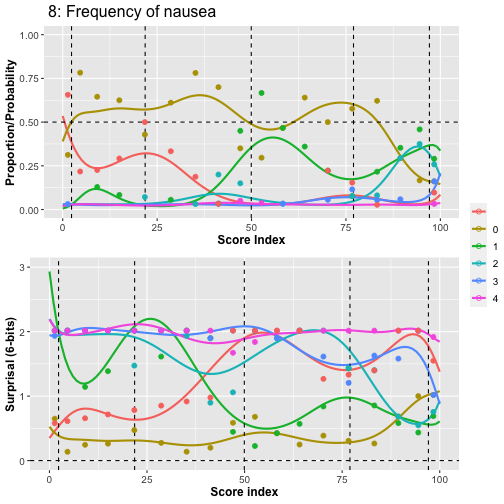Let's make a few observations on what we see in these two plots.

When probability goes up to one, surprise declines to zero, as we would expect. The probability a rating 0 is high and the surprisal is low if the respondent is in the bottom 25%, as we would expect. But, for some reason that is also the case if patient is near the 75% mark. Perhaps if the distress for other factors is that high, nausea considered of minor importance. Or, if one is that sick, nausea is relieved by a treatment. A mild distress rating of 1 appears at the 50% level. The probability of higher ratings is rare, and it seems that few patients at the upper end of the scale worry about this symptom. (We convert 6-bits into 2-bits by multiplying 3 5-bits by 2.585, the value of the logarithm to the base 2 of 6.)

It is the speed of an increase or decrease in the surprisal curve that is the fundamental signal that an examinee should be boosted up and dragged down, respectively, from a given position. The sharp increase in surprise for rating 0 at the 40% level signals that an examinee in that zone should be increased. Of course the examinee's final actual position will depend, not only on the five surprisal curves shown here, but also on those for the remaining 12 questions.

We call the rate of increase or decrease the “sensitivity” of an option. We have a specific plot for display this directly below.

The dots in the plot are the surprisal values for examinees in each of the 20 bins used in this analysis. The points are on the whole close their corresponding curves, suggesting that 473 examinees gives us a pretty fair idea of the shape of a surprisal or probability curve.

## Plot the probability density of percentile ranks

The density of the percentile ranks will no longer be a flat line. This is because examinees tend to cluster at various score levels, no matter how the score is defined. Certain groups of items will tend to be correctly answered by the middle performance folks and other by only the top performers. Among the weakest examinees, there will still be a subset of questions that they can handle. We want to see these clusters emerge.

``````ttllab     <- paste(titlestr,": percent rank", sep="")
scrrng     <- c(0,100)
theta_in   <- theta[theta > 0 & theta < 100]
indden10   <- TestGardener::scoreDensity(theta_in, scrrng, ttlstr=ttllab)
``````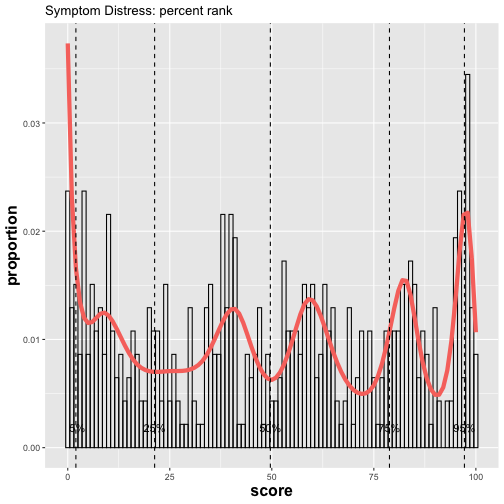Sure enough, there are four distinct clusters of score index values. Within each of these clusters there are strong similarities in examinee's choice patterns, whether right or wrong. We have only plotted score indices which are away from the two boundaries, because there are significant tendencies to have estimated score index values at 0 and 100.

## Compute expected test scores and plot their density function for all examinees

The expected score for an examinee are determined by his value `theta` for the question and by the score values assigned by the test designer to each option. We use a plotting interval defined by the range of the sum scores, but as a rule the density of expected scores is positive only over a shorter interval.

``````mu <- testscore(theta, WfdList, optList)
ttllab <- paste(titlestr,": expected score", sep="")
muden  <- TestGardener::scoreDensity(mu, SDS_dataList\$scrrng, ttlstr=ttllab)
``````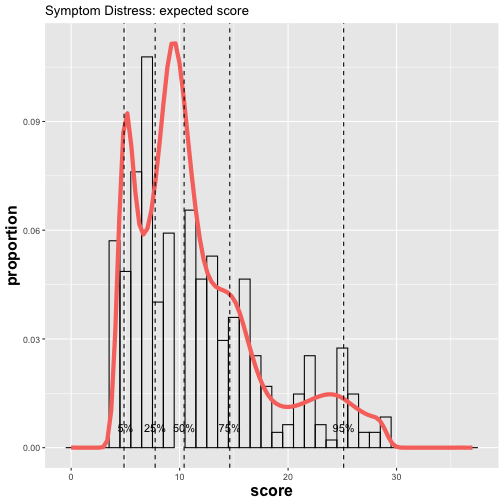The expected score distribution is much more compressed than the sum score ratings, with 10 being the favourite rating. But there is a cluster of ratings at the side of the distribution of 20, which corresponds to 6 ratings of 1 and 7 ratings of 2. Being in a hospital is never great, but it's nice to know that it is not unbearable, either. Or perhaps the nurses in Winnipeg, Manitoba where the data were collected are especially effective.

But at the top end, the best examinees lose heavily in average score terms. There are no average scores above 21, even though there are plenty of examinees with score indices of nearly or exactly 100. This effect is due to the fact that a few questions are faulty in the sense of not getting close to probability one or surprisal 0 at score index 100. We found, for example, that one question did not have any answer that could be judged correct by serious mathematical standards. And another question seemed to suffer from some teachers having overlooked covering the topic of “percent increase.”

## Plot expected test scores and expected test score over mesh

indfine <- seq(0,100,len=101) mufine <- TestGardener::testscore(indfine, WfdList, optList)

TestGardener::mu.plot(mufine, SDS_dataList\$scrrng, titlestr)

It is typical that lower expected test scores are above the diagonal line and higher ones below. The process of computing an expected score compresses the range of scores relative to that of the observed test scores.

## Compute the arc length of the test effort curve and plot the curve

We have 119 curves simultaneously changing location simultaneously in both probability and surprisal continua as the score index moves from 0 to 100. We can't visual such a thing, but we are right to think of this as a point moving along a curve in these two high dimensional spaces. In principle this curve has twists and turns in it, but we show below that they are not nearly as complex as one might imagine.

What we can study, and use in many ways, is the distance within the curve from its beginning to either any fixed point along it, or to its end. The curve is made especially useful because it can be shown that any smooth warping of the score index continuum will have no impact on the shape of this curve. Distance along the curve can be measured in bits, and a fixed change in bits has the same meaning at every point in the curve. The bit is a measure information, and we call this curve the test information curve.

The next analysis and display displays the length of the curve and how it changes relative to the score index associated with any point on the curve.

``````print(paste("Arc length =", round(arclength,2)))
#  "Arc length = 36.91"
TestGardener::ArcLength.plot(arclength, alfine, titlestr)
``````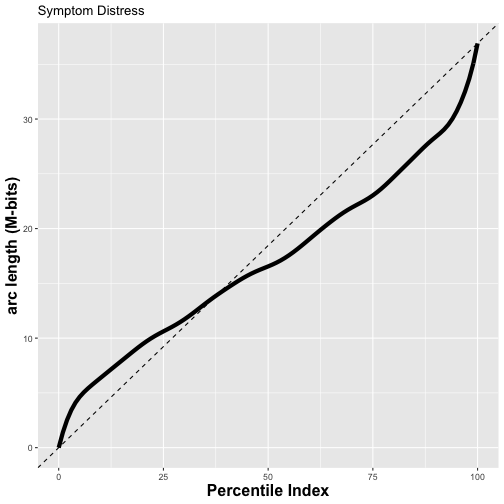The relationship is surprisingly linear with respect to the score index theta, except for some curvature near 0 and 100. We can say of the top examinees that they acquire nearly 69 2-bits of information represented in the test. That is, the probability of getting to the highest arclength is equivalent to tossing 69 heads in a row. (We convert 6-bits into 2-bits by multiplying 26.58 by 2.585]).

## Display test information curve projected into its first two principal components

The test information curve is in principle an object of dimension `Wdim`, but in most cases almost all of its shape can be seen in either two dimensions or three. Here we display it in two dimensions as defined by a functional principal component analysis.

``````Result <- TestGardener::Wpca.plot(arclength, WfdList, SDS_dataList\$Wdim, titlestr=titlestr)
``````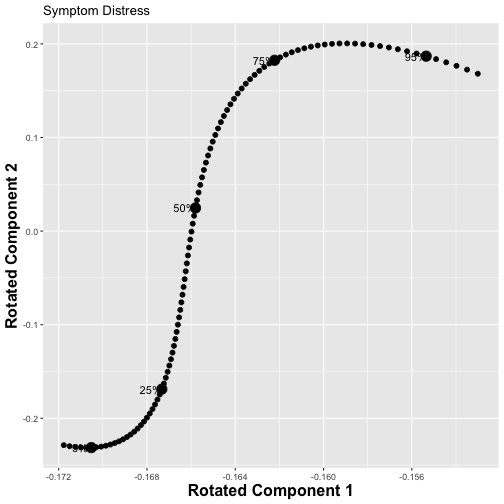There is a strong change in the direction of this curve at the 50% marker point. Given what we saw in the density plots, this seems to be the point where the patient experiences distress that would no longer be called normal.

## Display the sensitivity and power curves

The position of an examinee on the percentile rank continuum is directly determined by the rate of change or the derivative of the surprisal curve. An option becomes an important contributor to defining this position if it deviates strongly from zero on either side. This is just as true for wrong answers as it is for right answers. In fact, the estimated percentile rank value of an examinee is unaffected by what option is designated as correct. It is not rare that a question actually has two right answers, or even none, but such questions can still contribute to the percentile rank estimation.

``````TestGardener::Sensitivity.plot(WfdList, Qvec, SDS_dataList, titlestr=titlestr, plotindex=8)
``````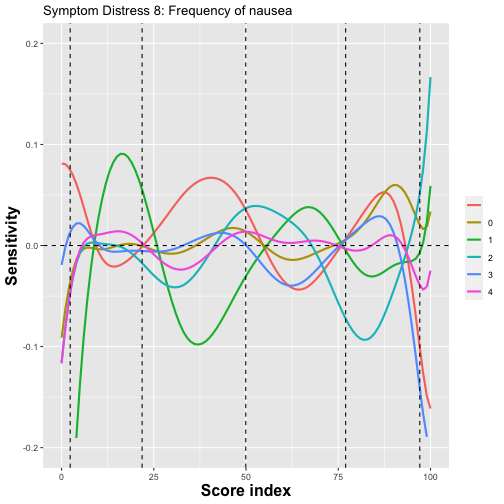The peaks and valleys in these curves are at the positions of the four clusters that we saw in the plot of the density of the score index theta.

The sensitivities of options can be collected together to provide a view of the overall strength of the contribution of a question to identifying an examinee's percentile rank. We do this by, at each point, adding the squares of the sensitivity values and taking the square root of the result. We call this the item power curve. Here is the power curve for question 9:

``````Result <- TestGardener::Power.plot(WfdList, Qvec, SDS_dataList, plotindex=9, height=0.3)
# Warning: Removed 1 rows containing missing values (geom_point).
``````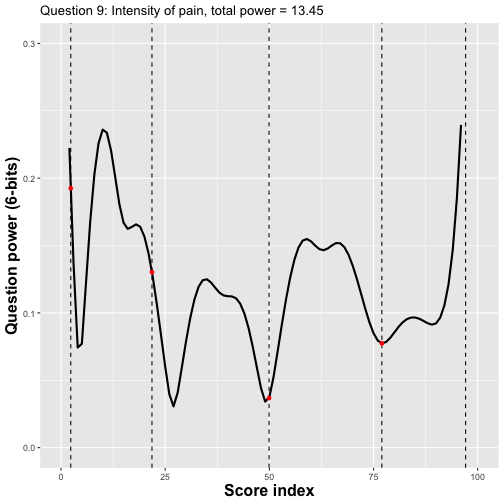We see only a small amount of power everywhere over the score index continuum except at the highest level of distress. The power integrated over the score index is among the lowest.

Now let's look at a question that has a great deal of power, question 8.

``````Result <- TestGardener::Power.plot(WfdList, Qvec, SDS_dataList, plotindex=8, height=0.3)
# Warning: Removed 1 rows containing missing values (geom_point).
``````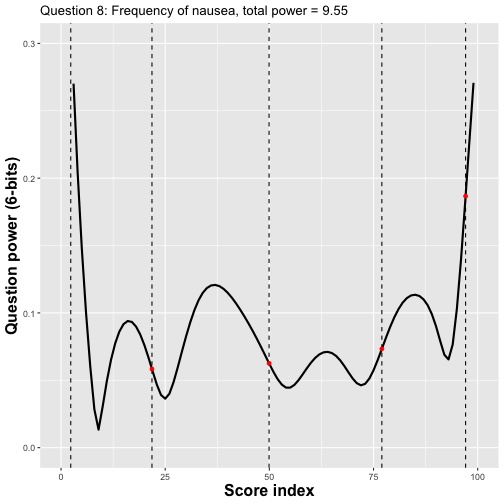##Investigate the status of score index estimate by plotting H(theta) and its derivatives

An optimal fitting criterion for modelling test data should have these features: (1) at the optimum value of theta fit values at neighbouring values should be larger than the optimum; (2) the first derivative of the fitting criterion should be zero or very nearly so, and (3) the second derivative should have a positive value.

But one should not automatically assume that there is a single unique best score index value that exists for an examinee or a ratings scale respondent. It's not at all rare that some sets of data display more than one minimum. After all, a person can know some portion of the information at an expert level but be terribly weak for other types of information. By tradition we don't like to tell people that there are two or more right answers to the question, “How much do you know?” But the reality is otherwise, and especially when the amount of data available is modest.

If an estimated score index seems inconsistent with the sum score value or is otherwise suspicious, the function Hfuns.plot() allows us to explore the shape of the fitting function H(theta) as well as that of its second derivative.

Here we produce these plots for the first five respondents. Each illustrates something important in the shapes of these curves.

``````TestGardener::Hfuns.plot(theta, WfdList, U, plotindex=1:5)
``````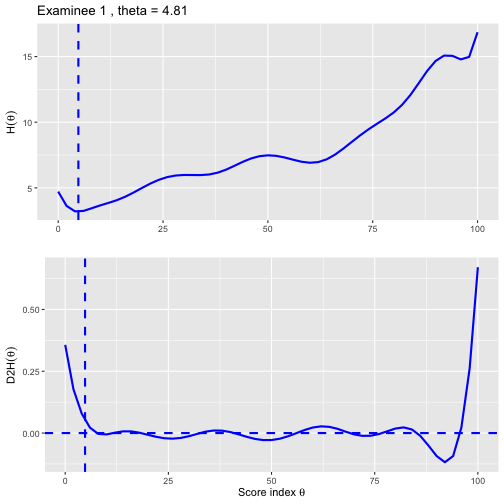``````# theta 1 . Press [enter] to continue
``````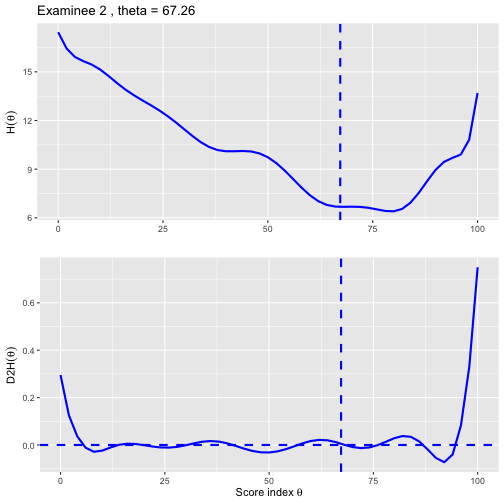``````# theta 2 . Press [enter] to continue
``````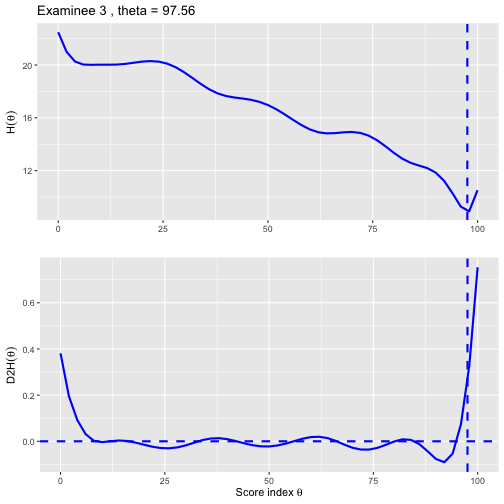``````# theta 3 . Press [enter] to continue
``````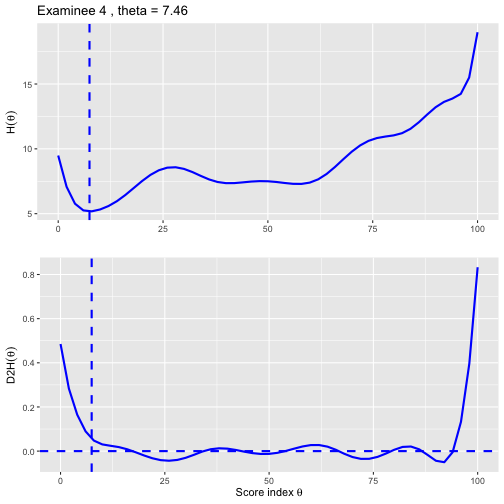``````# theta 4 . Press [enter] to continue
``````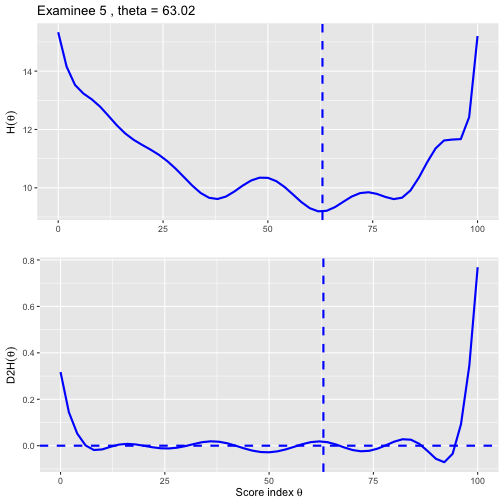``````# theta 5 . Press [enter] to continue
# []
``````